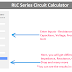In this RLC Series Circuit Calculator, you can calculate Impedance(Z), Inductive Reactance(XL), Capacitive Reactance(XC), Current Flow, Phase Angle, Power Factor, Reactive Power, Power Dissipation, and many other parameters.

RLC Series Circuit Calculator

## RLC Series Circuit Calculator

(Enter Your Values It will Automatically Generate the Result)

### How to Use This Calculator?

To use the above RLC Series Circuit Calculator, follow the below steps:

1. In the calculator, you will see input fields labeled for different parameters such as inductance (L), capacitance (C), resistance (R), voltage (U), and frequency (f). Enter the respective values you have for each parameter in the corresponding input fields. For each value, you can select the appropriate unit from the dropdown menus.

2. Once you input the values, the calculator will automatically generate results for various parameters of the RLC series circuit. You don't need to click any separate "Calculate" button. Also, the results will update automatically if you make any changes to the input values.

3. If you want to start over or clear the input values, you can click the "Reset" button.

4. The calculator will provide results for various parameters of the RLC series circuit.

5. You can choose the desired unit for each result from the dropdown menus provided next to the respective result.

### Formula

Here are the formulas, the calculator uses to calculate various parameters of the series RLC circuit,

Inductive Reactance (XL):

Formula: XL = 2 * π * f * L

Where XL is the inductive reactance in ohms (Ω), f is the frequency in hertz (Hz), and L is the inductance in henrys (H).

Capacitive Reactance (XC):

Formula: XC = 1 / (2 * π * f * C)

Where XC is the capacitive reactance in ohms (Ω), f is the frequency in hertz (Hz), and C is the capacitance in farads (F).

Impedance (Z):

Formula: Z = √(R^2 + (XL - XC)^2)

Where Z is the impedance in ohms (Ω), R is the resistance in ohms (Ω), XL is the inductive reactance in ohms (Ω), and XC is the capacitive reactance in ohms (Ω).

Current (I):

Formula: I = U / Z

Where I is the current in amperes (A), U is the voltage in volts (V), and Z is the impedance in ohms (Ω).

The voltage across Resistor (UR):

Formula: UR = R * I
Where UR is the voltage across the resistor in volts (V), R is the resistance in ohms (Ω), and I is the current in amperes (A).

The voltage across Inductor (UL):

Formula: UL = XL * I

Where UL is the voltage across the inductor in volts (V), XL is the inductive reactance in ohms (Ω), and I is the current in amperes (A).

The voltage across Capacitor (UC):

Formula: UC = XC * I

Where UC is the voltage across the capacitor in volts (V), XC is the capacitive reactance in ohms (Ω), and I is the current in amperes (A).

Power Dissipation through Resistor (QR):

Formula: QR = I * UR

Where QR is the power dissipation through the resistor in watts (W), I is the current in amperes (A), and UR is the voltage across the resistor in volts (V).

Power Dissipation through Inductor (QL):

Formula: QL = I * UL

Where QL is the power dissipation through the inductor in volt-amperes reactive (VAR), I is the current in amperes (A), and UL is the voltage across the inductor in volts (V).

Power Dissipation through Capacitor (QC):

Formula: QC = I * UC

Where QC is the power dissipation through the capacitor in volt-amperes reactive (VAR), I is the current in amperes (A), and UC is the voltage across the capacitor in volts (V).

Phase Angle (φ):

Formula: φ = arctan((XL - XC) / R)

Where φ is the phase angle in radians, XL is the inductive reactance in ohms (Ω), XC is the capacitive reactance in ohms (Ω), and R is the resistance in ohms (Ω).

Power Factor (PF):

Formula: PF = cos(φ)

Where PF is the power factor, and φ is the phase angle in radians.

Total Active Power (P):

Formula: P = U * I * cos(φ)

Where P is the total active power in watts (W), U is the voltage in volts (V), I is the current in amperes (A), and φ is the phase angle in radians.

Total Apparent Power (S):

Formula: S = U * I

Where S is the total apparent power in volt-amperes (VA), U is the voltage in volts (V), and I is the current in amperes (A).

Total Reactive Power (Q):

Formula: Q = U * I * sin(φ)

Where Q is the total reactive power in volt-amperes reactive (VAR), U is the voltage in volts (V), I is the current in amperes (A), and φ is the phase angle in radians.

Q Factor (Q):

Formula: Q = (1 / R) * √(L / C)

Where Q is the Q factor, R is the resistance in ohms (Ω), L is the inductance in henrys (H), and C is the capacitance in farads (F).

Thank you for visiting the Website. Keep visiting for more Updates.

RLC Series Circuit Calculator (Impedance, Reactance and More)Reviewed by Author on July 26, 2023 Rating: 5